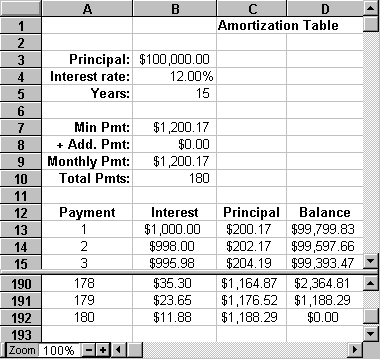## Concepts

### What is an amortization table?It's very easy to borrow money. It's much harder to determine the actual cost of borrowing. An amortization table is a document that will show you exactly how much you are paying each month for the privilege of borrowing.

There are four important components to an installment loan:

1. The principal or amount financed.
2. The interest rate or rate the lender is charging for the use of the money.
3. The term or amount of time for repayment.
4. The monthly payment which is determined by the first three items.

The key to understanding an amortization table is understanding the interest rate. The interest rate determines how much the lender is charging each much on the unpaid balance of the loan. The interest rate is usually specified in terms of an Annual Percentage Rate, or APR. To get the monthly percentage rate divide the APR by 12. As an example, if you borrowed \$100,000 at 12% APR, at the end of the first month you would owe \$1,000 (1% of \$100,000) in interest alone. If all you did was pay the interest cost each month you would be paying \$1,000 a month for infinite years!

Of course most loans are structured to be paid off in a certain number of years. To accomplish this the monthly payment must be greater than the monthly interest cost so that each month part of the payment goes toward the principal of the loan. As the principal is reduced the monthly interest cost is also reduced and with a fixed payment each month more of the payment is applied toward the principal of the loan. To continue the example above, if instead of \$1000 you paid \$1500 after the first month, \$1000 would go toward the interest and \$500 would go toward the principal. The balance of the loan for the second month is \$99,500. So, the interest owed for the second month is \$995 (1% of \$99,500). For the second payment \$995 is applied toward interest and \$505 is applied toward principal. This is progress. We have reduced the time to pay off the loan from infinite years to a little over nine years!

An amortization table is a document that shows for each payment of an installment loan the amount going toward interest and the amount going toward principal.

The figure above shows an example of an amortization table created with a spreadsheet. The split screen feature is being used to show the first and last three payments. The spreadsheet used to create this table is available for download.

As a final note, it should be pointed out that there are many different types of loans. The installment loan considered here is one of the most conventional. An amortization table can be applied to unconventional as well as conventional loans. In all cases it will show for each payment the amount going toward interest and the amount going toward principal.

Copyright 1996 by the Curators of the University of Missouri